SAT II Math I : Data Analysis and Statistics

Example Questions

Example Question #1 : Mode

What is the mode of 8, 7, 5, 4, 12, 13, 7, 2, 7?Explanation:

The mode is the number that is repeated the most within a set. In our case we see 7 repeated 3 times therefore our mode is 7

Example Question #82 : Data Analysis And Statistics

What's the mode?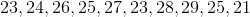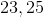Explanation:

Mode is the number occuring the most in the data set. By rearrangging in increasing order, we see thatandoccur twice. That will be our answers.

Example Question #83 : Data Analysis And Statistics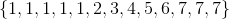Which value above would be the mode of this particular data set?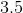Explanation:

The mode is the value that occurs the most times in one set.  In the given set that value iswhich occurs five times.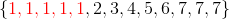Example Question #1 : Mode

A class of statistics students scored the following on their last test: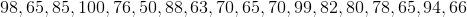.

Calculate the mode for this data set.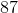Explanation:

The mode is the number that shows up more often than the others in a set of data.

If we put our data set in order we see:

98, 65, 85, 100, 76, 50, 88, 63, 70, 65, 70, 99, 82, 80, 78, 65, 94, 66

Becomes,

50, 63, 65, 65, 65, 66, 70, 70, 76, 78, 80, 82, 85, 88, 94, 98, 99, 100.

Therefore, 65 is the mode because it appears 3 times.

Example Question #2 : How To Find Mode

What is the mode of the following data set?

{9, 10, 4, 11, 7, 5, 15, 7, 2, 20, 11, 19, 7, 9}

7 and 9

2

11

7

9

7

Explanation:

Arrange the set in numerical order: {2, 4, 5, 7, 7, 7, 9, 9, 10, 11, 11, 15, 19, 20}.

You can then chart the frequency of each value.

Value (Frequency)

2 (1)

4 (1)

5 (1)

7 (3)

9 (2)

10 (1)

11 (2)

15 (1)

19 (1)

20 (1)

From this you can see the most common value, which is the mode, is 7.

Example Question #3 : Mode

A set comprises ten elements, which are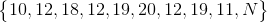wherestands for an unknown number.

What canbe set to in order to make the set bimodal?The set cannot be bimodal for any value of N.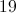Explanation:

12 appears in the set most frequently, appearing three times; 19 is second in frequency, appearing twice. 10, 11, 18, and 20 appear once each.

The only number we can setto to make two data values tie for appearing most frequently is 19; 12 and 19 would then each appear three times, and the others, once. Ifis set to any other number, 12 would remain the most frequent data value.

Example Question #14 : Mode

Find the mode of the set: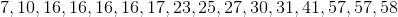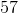Explanation:

The mode is the most repeated number in the set. To answer this, find the number that occurs the most number of times in the set.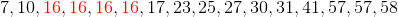For this problem that gives us the answer of 16 for the mode.

Example Question #321 : Basic Statistics

Find the mode of the set: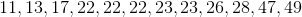Explanation:

The mode is the most repeated number in the set. To answer this, find the number that occurs the most number of times in the set.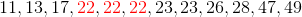For this problem that gives us the answer of 22 for the mode.

Example Question #16 : Mode

Find the mode of the set: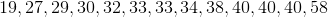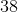Explanation:

The mode is the most repeated number in the set. To answer this, find the number that occurs the most number of times in the set.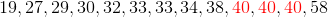For this problem that gives us the answer of 40 for the mode.

Example Question #17 : Mode

Find the mode of the following data set: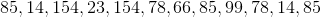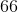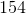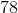Explanation:

Find the mode of the following data set:The mode is the number which appears most in a number set. In this case, we have a few duplicates, but only one triple: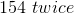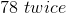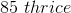Therefore, it must be 85, because 85 is the only one which appears more than twice!